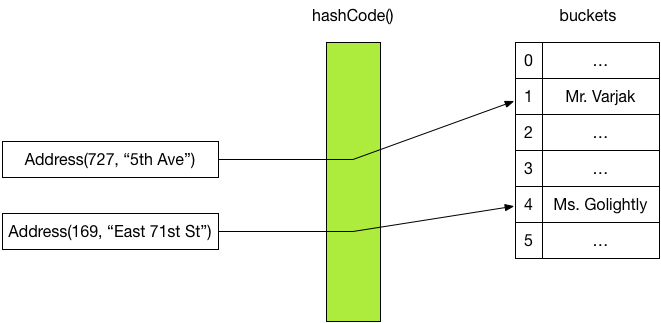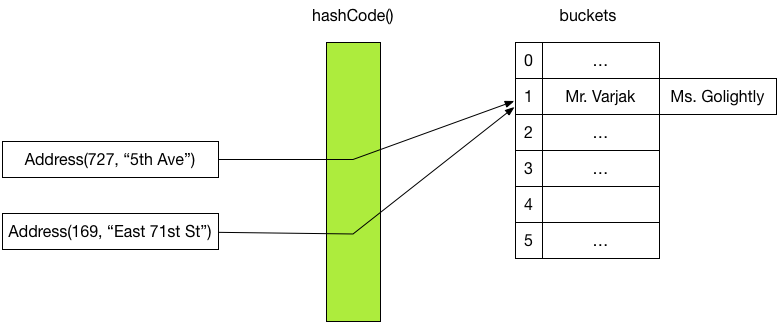# equals() and hashCode()

## July 3, 2015

As a programmer new to Java one of the most common pieces of advice is to “always override both `equals()` and `hashCode()`”. In this post we will explore this piece of advice further and investigate what can go wrong if we do not follow it.

## equals()

The `equals()` method is self-explanatory: it checks whether two objects are equal returns `true` if they are, and false otherwise. More formally, “the equals method implements an equivalence relation on non-null object references” 1.

The default implementation that Java’s `Object` base class provides simply checks whether the two objects being compared are one and the same object:

``````public boolean equals(Object other) {
return this == other;
}``````

However, once we build more complex classes it can often be useful to override the method to consider objects with similar properties “equal”. For example, in an `Address` class, we may want to consider two instances with the same street and number the same address:

``````class Address {
private int number;
private String street;

...

@Override
public boolean equals(Object other) {
return false;
}

}
}``````

Overriding `equals()` can be particularly beneficial when dealing with Java’s collections. If we wish to check if an `Address` is contained in a list of addresses, the `contains()` method uses `equals()` method:

``````public static void main(String args[]) {

...

// will be executed
}
}``````

Here, the block contained inside the `if`-statement will be executed even though we compare two separate objects — the program will print “Address exists!”. This is thanks to the new implementation of the `equals()` method.

## hashCode()

So far, we have not been required to also override the `hashCode()` method in order to observe the desired behaviour of our code.

Without implementing `hashCode()`, let us now consider a similar address example, in which we use a `Map` to associate addresses with the name of their primary residents:

``````public static void main(String args[]) {
aNames.put(new Address(727, "5th Ave"), "Mr. Varjak");
aNames.put(
new Address(169, "East 71st St"), "Ms. Golightly"
);

...

}
}``````

Clearly, in this example, Ms. Golightly lives at 169 East 71st St. However, execution of this program does not result in any output on the screen. What is going on?

As it turns out, the Java `HashMap` implementation uses so-called hashing to efficiently look up values for specific keys. Instead of keeping a list of all of the map entries and searching the entire list for a specific key, `HashMap` makes use of a hash function to transform the key of an entry into an integer. This integer value can then be used to index into a table of values 2. The following diagram illustrates this. The `Address` instance is converted into an integer using `hashCode()` — which is Java’s hash function — which is then used to index into a table of names. To find an element in the hashMap by key, all we have to do is therefore call its `hashCode()` method to find its value, making this an efficient operation. Notice that when doing this, `equals()` is not called.Similarly, looking up values by key in a `HashMap` is also done by converting the search key to an integer via its `hashCode()` method.

However, Java’s default implementation of the `hashCode()` method maps the specific object’s address to an address. This means that not overriding `hashCode()` can lead to interesting consequences. For example, adding the same address–name pair to the map twice can lead to the following memory organisation, in which the pair has been recorded twice:Therefore, keys that we consider equal should also hash to the same integer value. Thus, when overriding `equals()` you should also override `hashCode()`.

## equals() in HashMap

In our examples so far, we have always either used `equals()` or `hashCode()` but never both. You may therefore ask whether it may be sufficient to implement `hashCode()` only in some cases. Apart from the fact that tying the logic of your program so closely to the implementation is a bad idea, there are cases when both `hashCode()` and `equals()` are used together.

In fact, two objects that are not equal according to `equals()` are allowed to share the same hash code. This means that it is possible for two different addresses to end up in the same bucket.`HashMap`’s buckets therefore do not store values directly but instead a collection of values. When a search key hashes to a particular bucket, the `equals()` method is then used to determine which of the objects in that bucket should be returned, if any.

## Conclusions

We have seen that when overriding `equals()` you should also always override `hashCode()` and vice-versa. Two objects that are considered equal should always hash to the same hash code. However, two objects that hash to the same bucket do not necessarily have to be considered equal.

1. More information on hashing and hash tables can be found under https://en.wikipedia.org/wiki/Hash_table